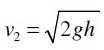We were discussing the basic difference between orifice and mouthpiececlassification of orifices and mouthpieces and also advantages and disadvantages of orifices in the subject of fluid mechanics, in our recent posts. Now we will go ahead to find out the expression for flow through an orifice.

First we will see here the basic concept of an orifice and after that we will find out here the expression for flow through an orifice with the help of this post. So let us come to the main topic, without wasting your time.

### Orifice

An orifice is basically a small opening of any cross-section such as triangular, square or rectangular on the side or at the bottom of tank, through which a fluid is flowing. Orifice is basically used in order to determine the rate of flow of fluid.

As we have discussed above that orifice will be a small opening of any cross-section, hence flow through the orifice will be very small.

### Flow through an orifice

Let us consider one tank with a circular orifice fitted at one side of the tank as displayed here in following figure.

Liquid flowing through the orifice is developing a liquid jet whose cross-sectional area is smaller than the cross-sectional area of the circular orifice. Area of liquid jet is decreasing and area is minimum at section CC.

Section CC will be approximately at a distance of half of diameter of the circular orifice. At section CC, the streamlines are straight and parallel with each other and perpendicular to the plane of the orifice. This section CC will be termed as Vena-contracta.

Beyond the section CC, liquid jet diverges and will be attracted towards the downward direction due to gravity.
Image: Tank with a circular orifice

Let us consider that h is the head of the liquid above the centre of orifice.

Let us consider two points 1 and 2 as displayed in above figure. Point 1 is displayed inside the tank and point 2 is shown at the Vena-contracta.

### Assumption

Let us consider that flow is steady and at a constant differential head h.
p1 = Pressure at point 1
v1 = Velocity of fluid at point 1
p2 = Pressure at point 2
v2 = Velocity of fluid at point 2

Now we will apply the Bernoulli’s equation at point 1 and 2.
Area of tank is quite large as compared with area of liquid jet and therefore v1 will be very small as compared with v2Therefore above expression for theoretical velocity could be re-expressed as mentioned here.We must note it here that this is the theoretical velocity and actual velocity will be less than this value.
We will see various types of hydraulic co-efficients, in the subject of fluid mechanics, in our next post.

Do you have any suggestions? Please write in comment box.

### Reference:

Fluid Mechanics, By R. K. Bansal